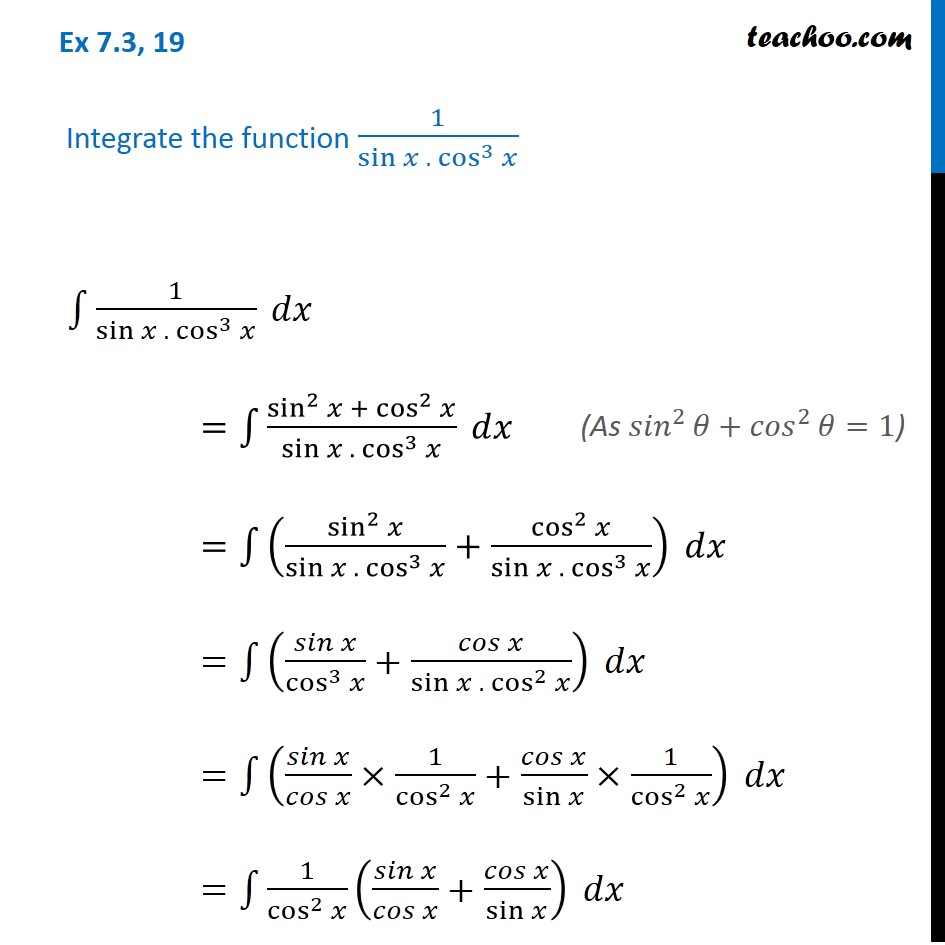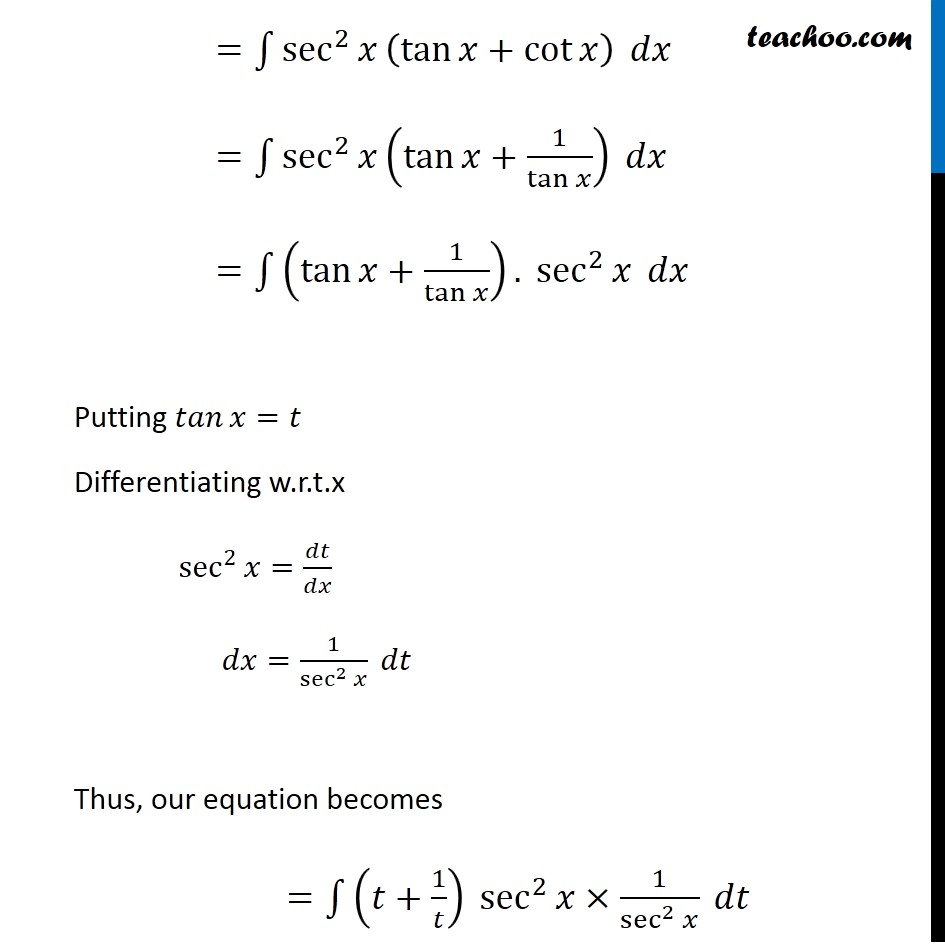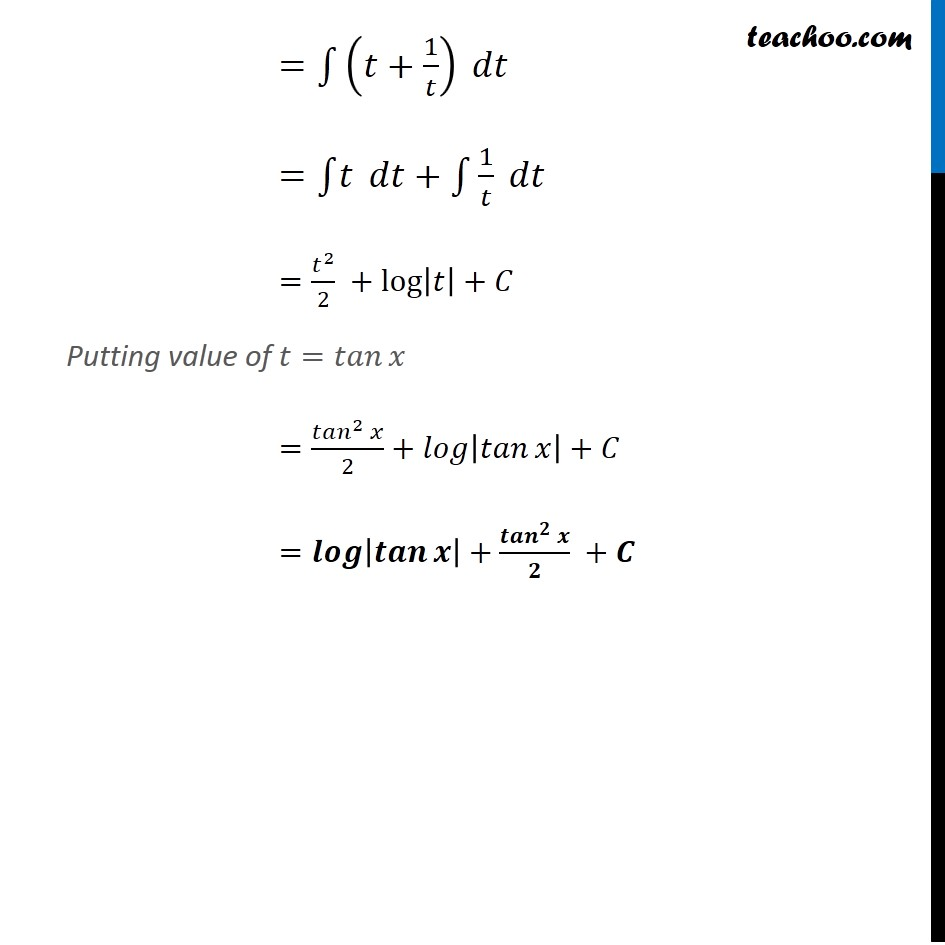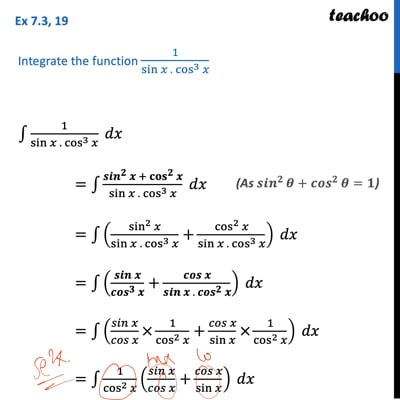Ex 7.3

Chapter 7 Class 12 Integrals
Serial order wiseThis video is only available for Teachoo black users

Get live Maths 1-on-1 Classs - Class 6 to 12

### Transcript

Ex 7.3, 19 Integrate the function 1/(sin⁡𝑥 . cos^3⁡𝑥 ) ∫1▒1/(sin⁡𝑥 . cos^3⁡𝑥 ) 𝑑𝑥 =∫1▒(sin^2⁡𝑥 + cos^2⁡𝑥)/(sin⁡𝑥 . cos^3⁡𝑥 ) 𝑑𝑥 =∫1▒(sin^2⁡𝑥/(sin⁡𝑥 . cos^3⁡𝑥 )+cos^2⁡𝑥/(sin⁡𝑥 . cos^3⁡𝑥 )) 𝑑𝑥 =∫1▒(𝑠𝑖𝑛⁡𝑥/cos^3⁡𝑥 +𝑐𝑜𝑠⁡𝑥/(sin⁡𝑥 . cos^2⁡𝑥 )) 𝑑𝑥 =∫1▒(𝑠𝑖𝑛⁡𝑥/𝑐𝑜𝑠⁡𝑥 ×1/cos^2⁡𝑥 +𝑐𝑜𝑠⁡𝑥/sin⁡𝑥 ×1/cos^2⁡𝑥 ) 𝑑𝑥 =∫1▒〖1/cos^2⁡𝑥 (𝑠𝑖𝑛⁡𝑥/𝑐𝑜𝑠⁡𝑥 +𝑐𝑜𝑠⁡𝑥/sin⁡𝑥 ) 〗 𝑑𝑥 (As 〖𝑠𝑖𝑛〗^2⁡𝜃+〖𝑐𝑜𝑠〗^2⁡𝜃=1) =∫1▒〖sec^2⁡𝑥 (tan⁡𝑥+cot⁡𝑥 ) 〗 𝑑𝑥 =∫1▒〖sec^2⁡𝑥 (tan⁡𝑥+1/tan⁡𝑥 ) 〗 𝑑𝑥 =∫1▒〖(tan⁡𝑥+1/tan⁡𝑥 ). sec^2⁡𝑥 〗 𝑑𝑥 Putting 𝑡𝑎𝑛⁡𝑥=𝑡 Differentiating w.r.t.x sec^2⁡𝑥=𝑑𝑡/𝑑𝑥 𝑑𝑥=1/sec^2⁡𝑥 𝑑𝑡 Thus, our equation becomes =∫1▒〖(𝑡+1/𝑡) sec^2⁡𝑥×1/sec^2⁡𝑥 〗 𝑑𝑡 =∫1▒(𝑡+1/𝑡) 𝑑𝑡 =∫1▒𝑡 𝑑𝑡+∫1▒1/𝑡 𝑑𝑡 =𝑡^2/2 +log⁡|𝑡|+𝐶 Putting value of 𝑡=𝑡𝑎𝑛⁡𝑥 =(〖𝑡𝑎𝑛〗^2 𝑥)/2+𝑙𝑜𝑔⁡|𝑡𝑎𝑛⁡𝑥 |+𝐶 =𝒍𝒐𝒈⁡|𝒕𝒂𝒏⁡𝒙 |+(〖𝒕𝒂𝒏〗^𝟐 𝒙)/𝟐 +𝑪